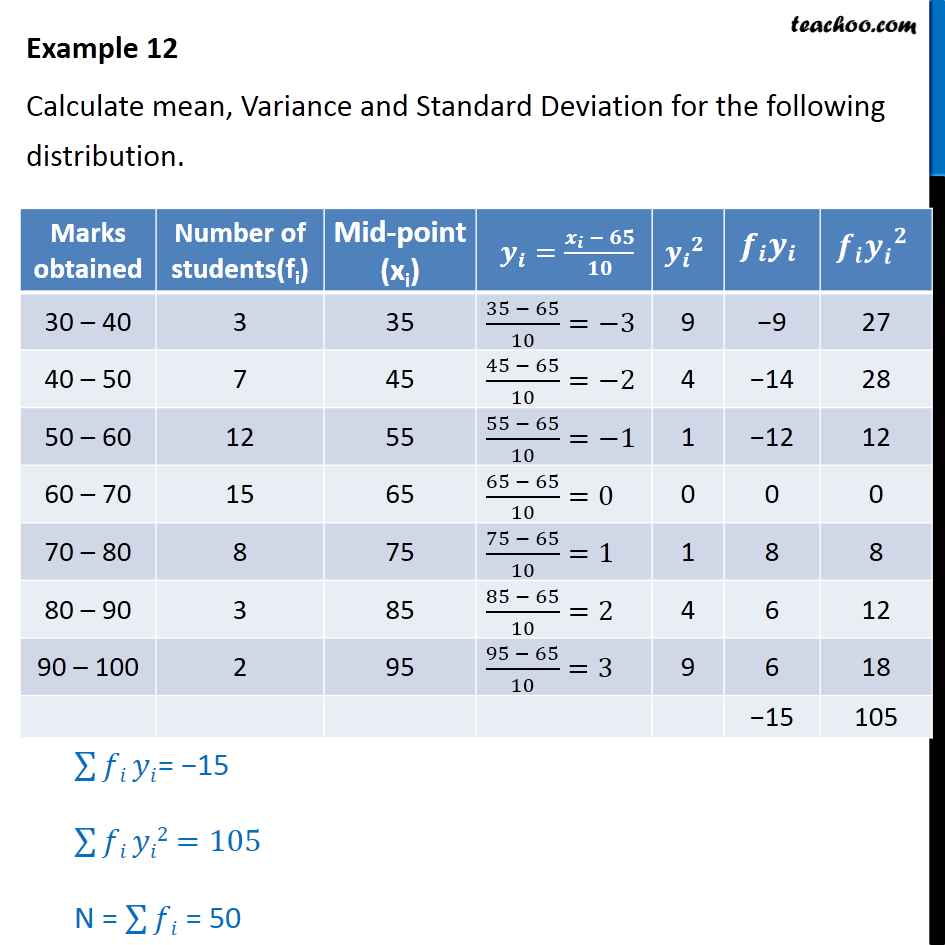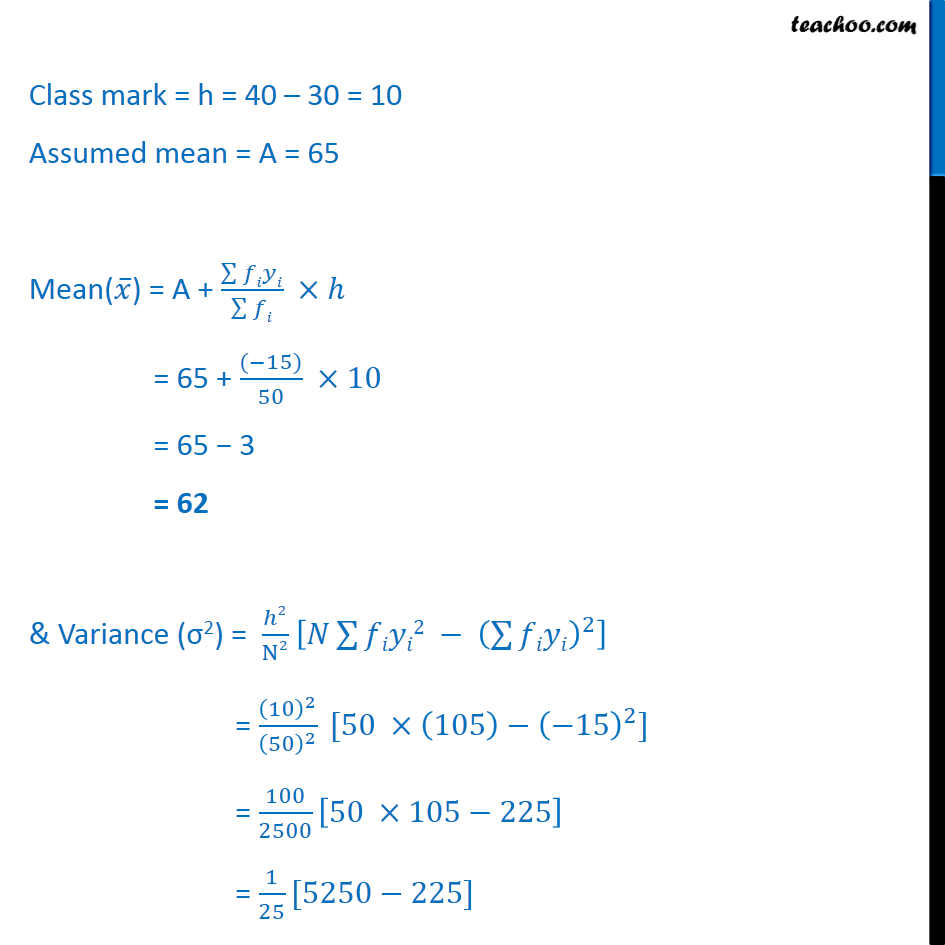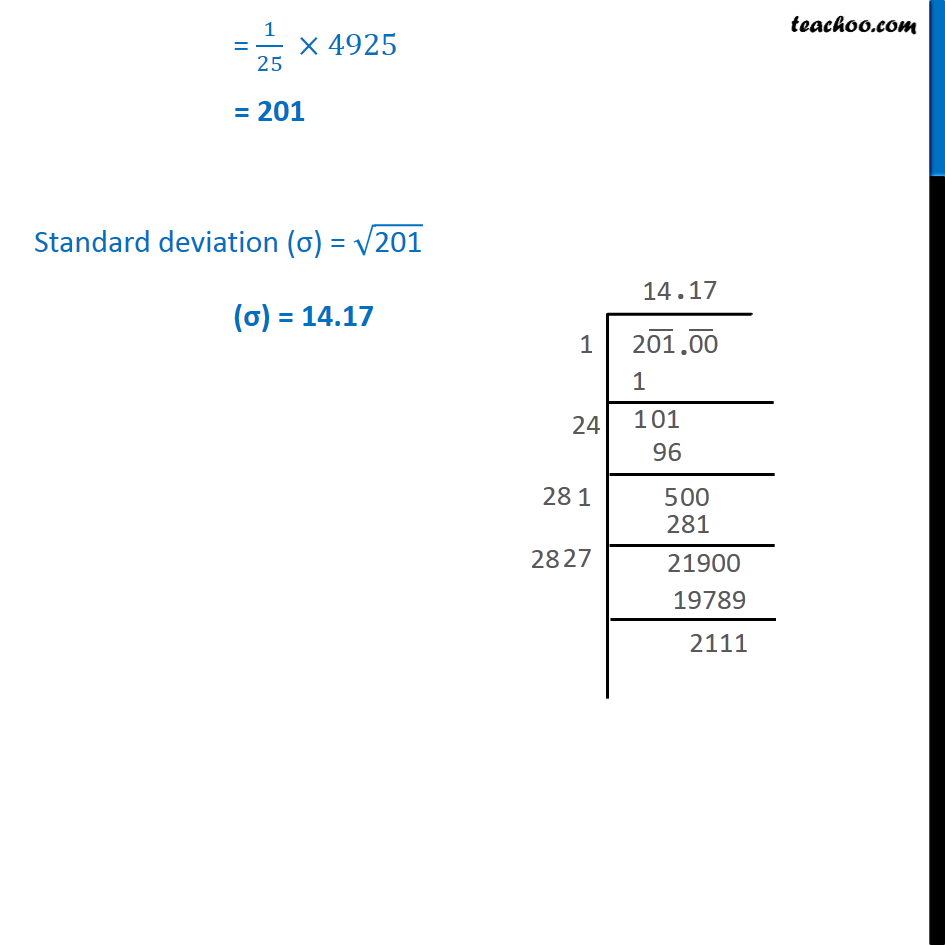Examples

Chapter 13 Class 11 Statistics
Serial order wiseLearn in your speed, with individual attention - Teachoo Maths 1-on-1 Class

### Transcript

Example 12 Calculate mean, Variance and Standard Deviation for the following distribution. 1﷮25﷯ ×4925 = 201 Finding Root of 201 Standard deviation (σ) = ﷮201﷯ (σ) = 14.17